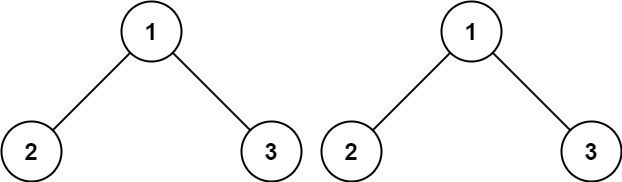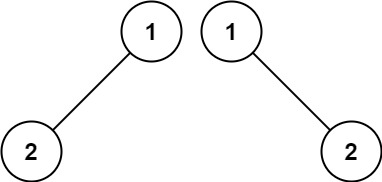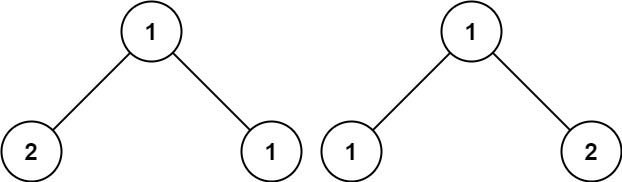100. Same Tree
Easy
9.1K
182

Given the roots of two binary trees `p` and `q`, write a function to check if they are the same or not.

Two binary trees are considered the same if they are structurally identical, and the nodes have the same value.

Example 1:```Input: p = [1,2,3], q = [1,2,3]
Output: true
```

Example 2:```Input: p = [1,2], q = [1,null,2]
Output: false
```

Example 3:```Input: p = [1,2,1], q = [1,1,2]
Output: false
```

Constraints:

• The number of nodes in both trees is in the range `[0, 100]`.
• `-104 <= Node.val <= 104`
Accepted
1.5M
Submissions
2.5M
Acceptance Rate
58.1%

Seen this question in a real interview before?
1/4
Yes
No

Discussion (0)

Related Topics